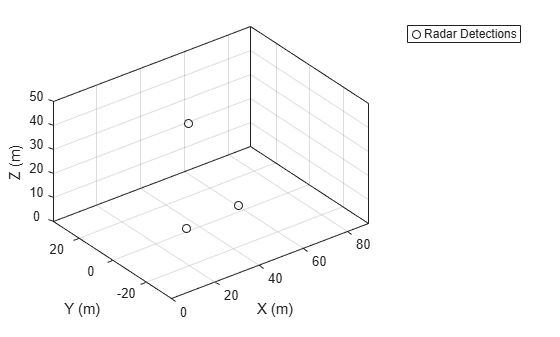# theaterPlot

Plot objects, detections, and tracks in Scenario

Since R2018b

## Description

The `theaterPlot` object is used to display a plot of a `trackingScenario`. This type of plot can be used with sensors capable of detecting objects.

To display aspects of a scenario on a theater plot:

1. Create a `theaterPlot` object.

2. Create plotters for the aspects of the scenario that you want to plot.

3. Use the plotters with their corresponding plot functions to display those aspects on the theater plot.

This table shows the plotter functions to use based on the scenario aspect that you want to plot.

## Creation

### Syntax

``tp = theaterPlot``
``tp = theaterPlot(Name,Value)``

### Description

````tp = theaterPlot` creates a theater plot in a new figure.```

example

````tp = theaterPlot(Name,Value)` creates a theater plot in a new figure with optional input Properties specified by one or more `Name,Value` pair arguments. Properties can be specified in any order as `Name1,Value1,...,NameN,ValueN`. Enclose each property name in quotes.```

## Properties

expand all

Parent axes, specified as a `theaterPlot` handle. If you do not specify `Parent`, then `theaterPlot` creates axes in a new figure.

Plotters created for the theater plot, specified as an array of plotter objects.

Limits of the x-axis, specified as a two-element row vector, [x1,x2]. The values x1 and x2 are the lower and upper limits, respectively, for the theater plot display. If you do not specify the limits, then the default values for the `Parent` property are used. See Orientation, Position, and Coordinate Convention for coordinate system definitions.

Data Types: `double`

Limits of the y-axis, specified as a two-element row vector, [y1,y2]. The values y1 and y2 are the lower and upper limits, respectively, for the theater plot display. If you do not specify the limits, then the default values for the `Parent` property are used. See Orientation, Position, and Coordinate Convention for coordinate system definitions.

Data Types: `double`

Limits of the z-axis, specified as a two-element row vector, [z1,z2]. The values z1 and z2 are the lower and upper limits, respectively, for the theater plot display. If you do not specify the limits, then the default values for the `Parent` property are used. See Orientation, Position, and Coordinate Convention for coordinate system definitions.

Data Types: `double`

Unit of each axes, specified as a three-element string array. Each element must be `"m"` or `"km"`

Data Types: `string`

## Object Functions

expand all

 `coveragePlotter` Create coverage plotter `detectionPlotter` Create detection plotter `orientationPlotter` Create orientation plotter `platformPlotter` Create platform plotter `trackPlotter` Create track plotter `trajectoryPlotter` Create trajectory plotter `surfacePlotter` Create surface plotter
 `plotCoverage` Plot set of coverages in theater coverage plotter `plotDetection` Plot set of detections in theater detection plotter `plotOrientation` Plot set of orientations in orientation plotter `plotPlatform` Plot set of platforms in platform plotter `plotTrack` Plot set of tracks in theater track plotter `plotTrajectory` Plot set of trajectories in trajectory plotter `plotSurface` Plot surfaces in theater surface plotter
 `clearData` Clear data from specific plotter of theater plot `clearPlotterData` Clear plotter data from theater plot `findPlotter` Return array of plotters associated with theater plot

## Examples

collapse all

Create a theater plot.

`tp = theaterPlot('XLim',[0 90],'YLim',[-35 35],'ZLim',[0 50]);`

Display radar detections with coordinates at $\left(30,\text{\hspace{0.17em}}-5,\text{\hspace{0.17em}}5\right)$, $\left(50,\text{\hspace{0.17em}}-10,\text{\hspace{0.17em}}10\right)$, and $\left(40,\text{\hspace{0.17em}}7,\text{\hspace{0.17em}}40\right)$. Set the view so that you are looking on the yz-plane. Confirm the y- and z-coordinates of the radar detections are correct.

```radarPlotter = detectionPlotter(tp,'DisplayName','Radar Detections'); plotDetection(radarPlotter, [30 -5 5; 50 -10 10; 40 7 40]) grid on view(90,0)```The view can be changed by opening the plot in a figure window and selecting Tools > Rotate 3D in the figure menu.

## Limitations

You cannot use the rectangle-zoom feature in the `theaterPlot` figure.

## Version History

Introduced in R2018b